Скачать презентацию Root Locus Method Root Locus Motivation

26b509efe28ab33765c79fbb217a8dcc.ppt

• Количество слайдов: 33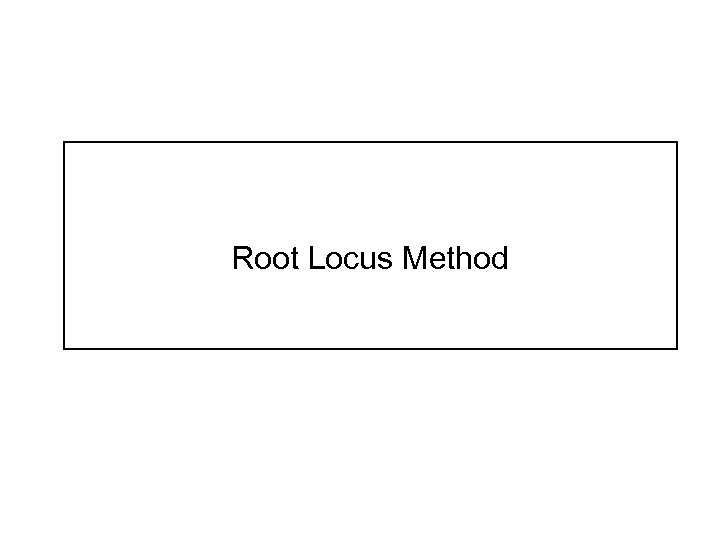Root Locus Method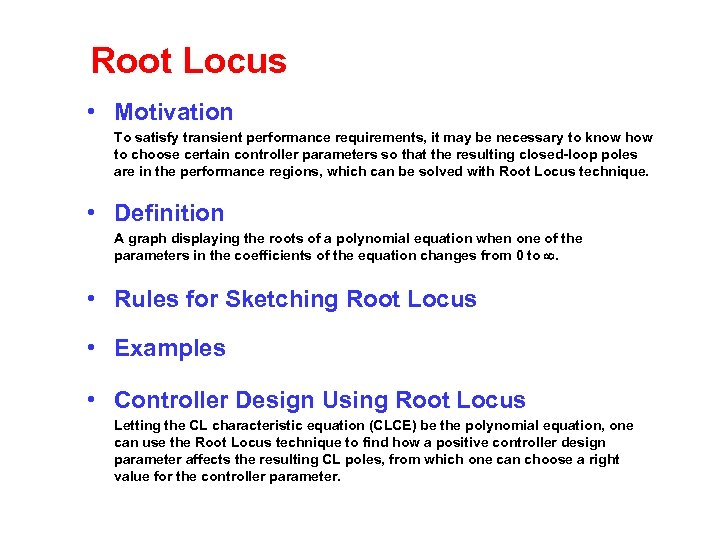Root Locus • Motivation To satisfy transient performance requirements, it may be necessary to know how to choose certain controller parameters so that the resulting closed-loop poles are in the performance regions, which can be solved with Root Locus technique. • Definition A graph displaying the roots of a polynomial equation when one of the parameters in the coefficients of the equation changes from 0 to . • Rules for Sketching Root Locus • Examples • Controller Design Using Root Locus Letting the CL characteristic equation (CLCE) be the polynomial equation, one can use the Root Locus technique to find how a positive controller design parameter affects the resulting CL poles, from which one can choose a right value for the controller parameter.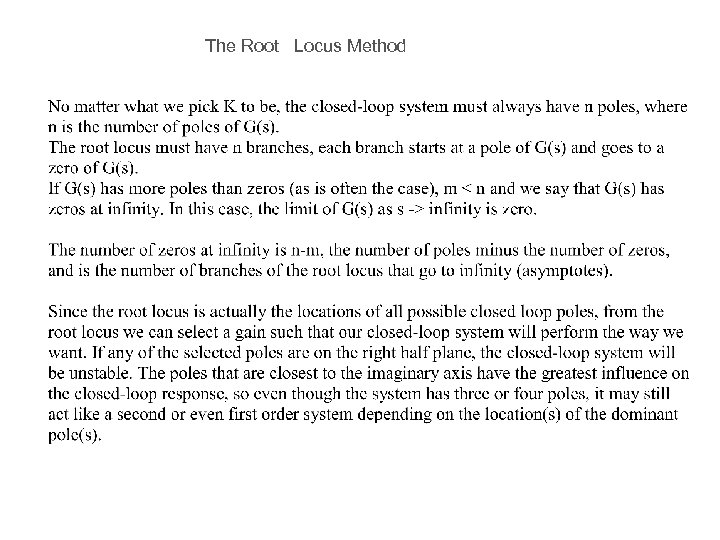The Root Locus Method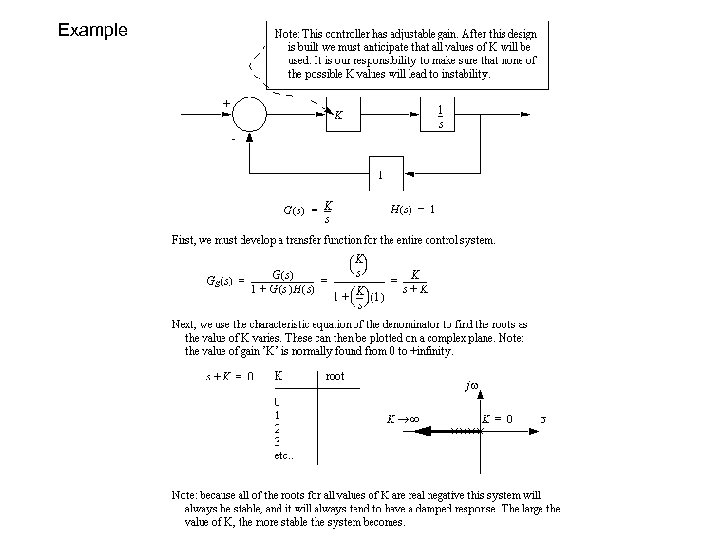Example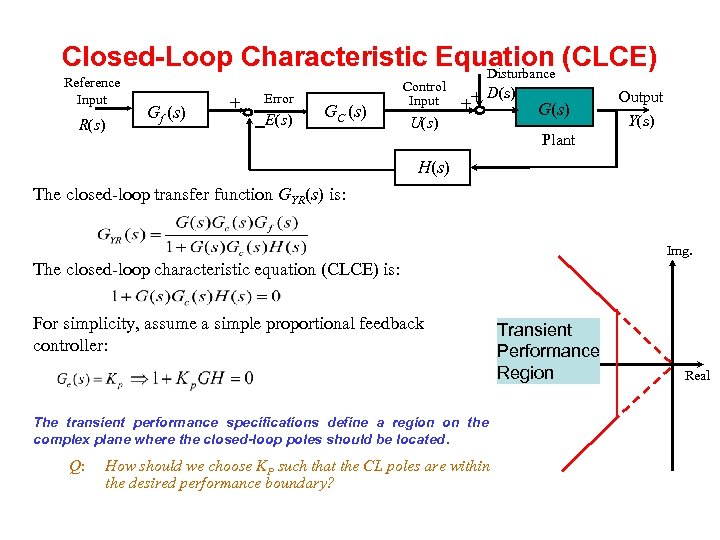Closed-Loop Characteristic Equation (CLCE) Disturbance Reference Input R(s) Gf (s) + Error -E(s) GC (s) Control Input U(s) D(s) ++ G(s) Output Y(s) Plant H(s) The closed-loop transfer function GYR(s) is: Img. The closed-loop characteristic equation (CLCE) is: For simplicity, assume a simple proportional feedback controller: The transient performance specifications define a region on the complex plane where the closed-loop poles should be located. Q: How should we choose KP such that the CL poles are within the desired performance boundary? Transient Performance Region Real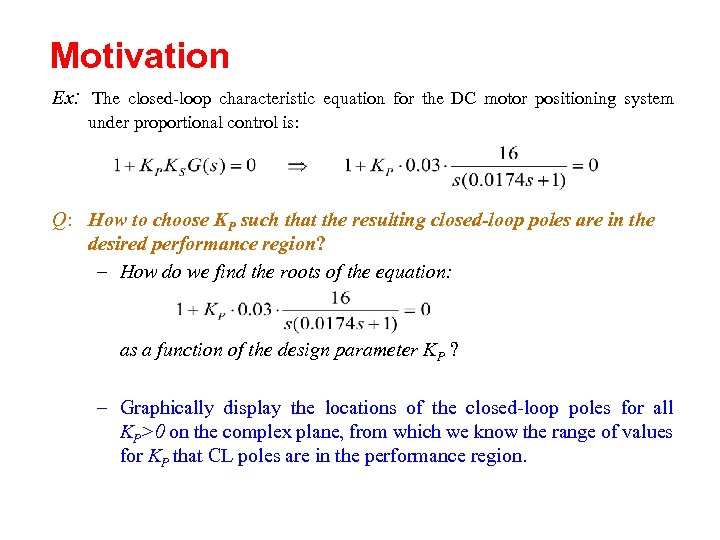Motivation Ex: The closed-loop characteristic equation for the DC motor positioning system under proportional control is: Q: How to choose KP such that the resulting closed-loop poles are in the desired performance region? – How do we find the roots of the equation: as a function of the design parameter KP ? – Graphically display the locations of the closed-loop poles for all KP>0 on the complex plane, from which we know the range of values for KP that CL poles are in the performance region.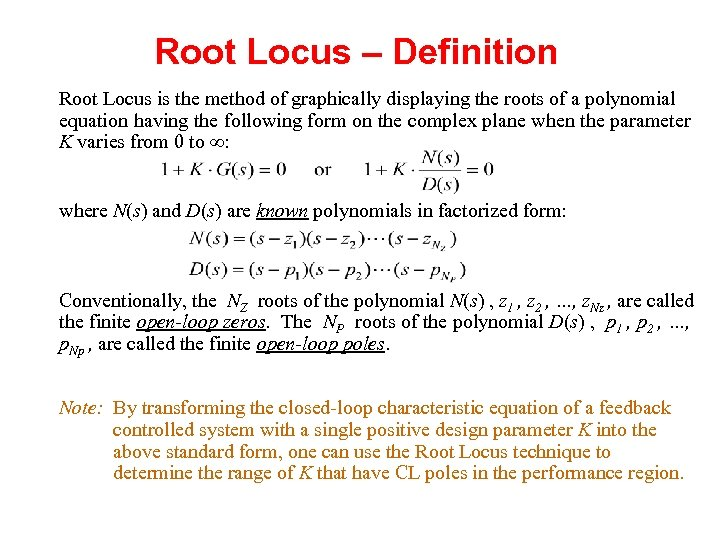Root Locus – Definition Root Locus is the method of graphically displaying the roots of a polynomial equation having the following form on the complex plane when the parameter K varies from 0 to : where N(s) and D(s) are known polynomials in factorized form: Conventionally, the NZ roots of the polynomial N(s) , z 1 , z 2 , …, z. Nz , are called the finite open-loop zeros. The NP roots of the polynomial D(s) , p 1 , p 2 , …, p. Np , are called the finite open-loop poles. Note: By transforming the closed-loop characteristic equation of a feedback controlled system with a single positive design parameter K into the above standard form, one can use the Root Locus technique to determine the range of K that have CL poles in the performance region.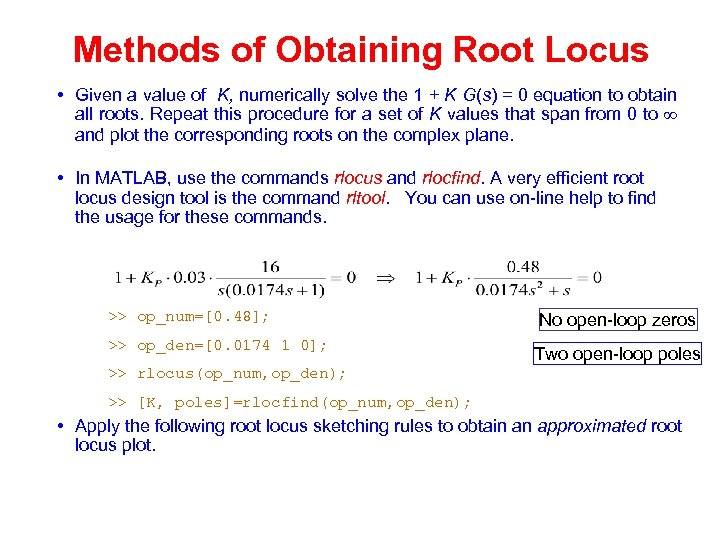Methods of Obtaining Root Locus • Given a value of K, numerically solve the 1 + K G(s) = 0 equation to obtain all roots. Repeat this procedure for a set of K values that span from 0 to and plot the corresponding roots on the complex plane. • In MATLAB, use the commands rlocus and rlocfind. A very efficient root locus design tool is the command rltool. You can use on-line help to find the usage for these commands. >> op_num=[0. 48]; >> op_den=[0. 0174 1 0]; No open-loop zeros Two open-loop poles >> rlocus(op_num, op_den); >> [K, poles]=rlocfind(op_num, op_den); • Apply the following root locus sketching rules to obtain an approximated root locus plot.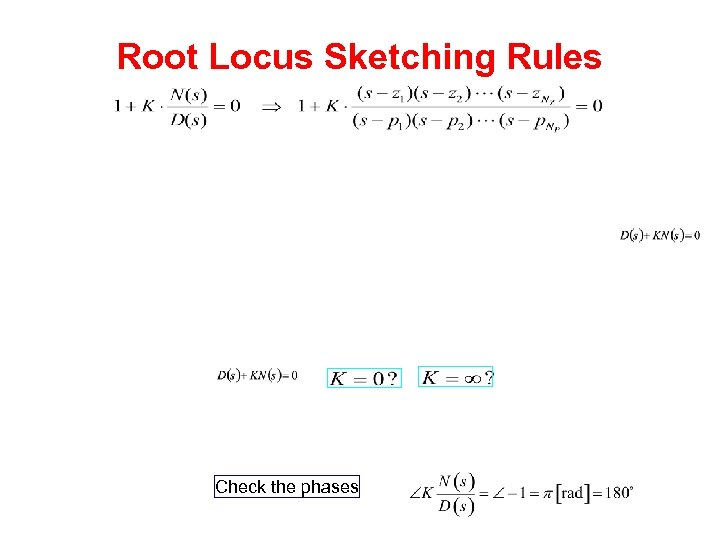Root Locus Sketching Rules Check the phases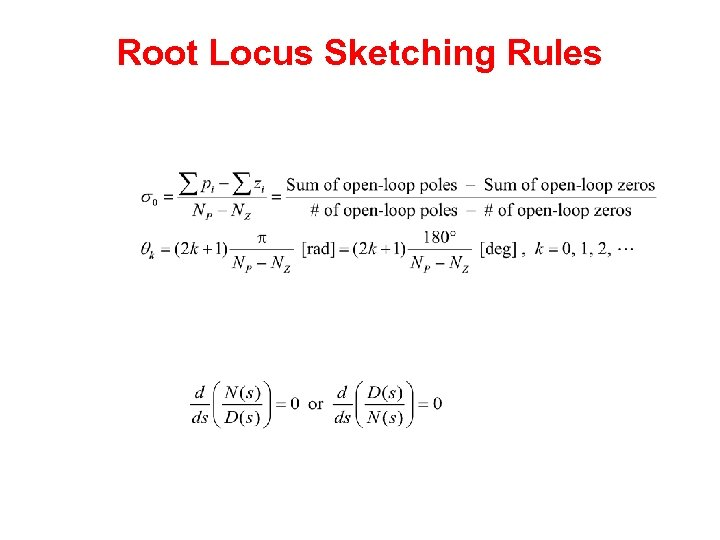Root Locus Sketching Rules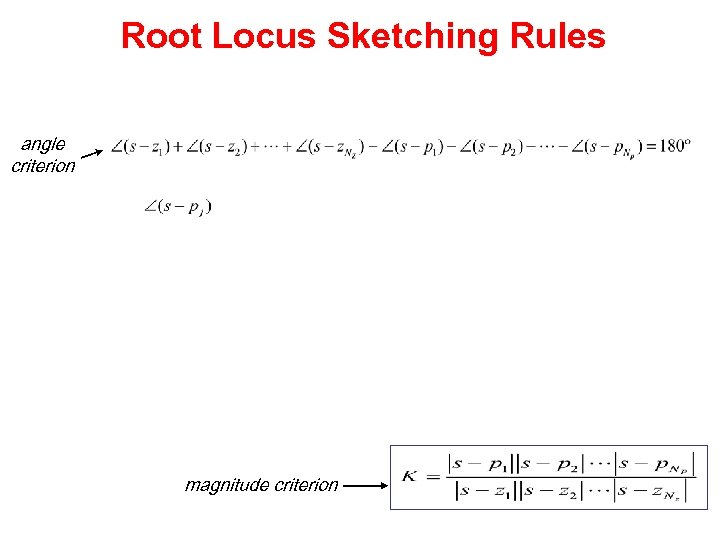Root Locus Sketching Rules angle criterion magnitude criterion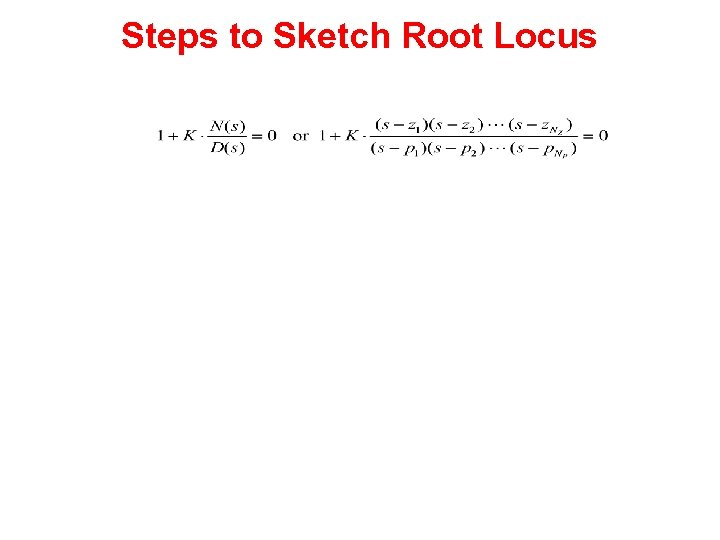Steps to Sketch Root Locus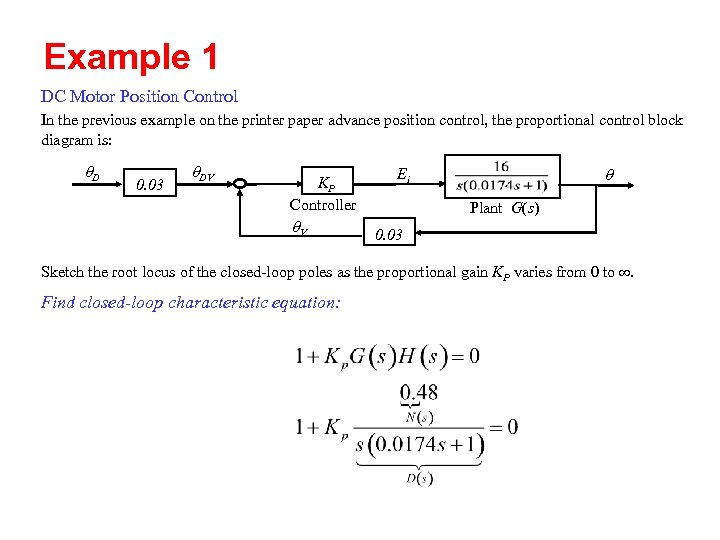Example 1 DC Motor Position Control In the previous example on the printer paper advance position control, the proportional control block diagram is: q. D 0. 03 q. DV KP Controller q. V q Ei Plant G(s) 0. 03 Sketch the root locus of the closed-loop poles as the proportional gain KP varies from 0 to . Find closed-loop characteristic equation: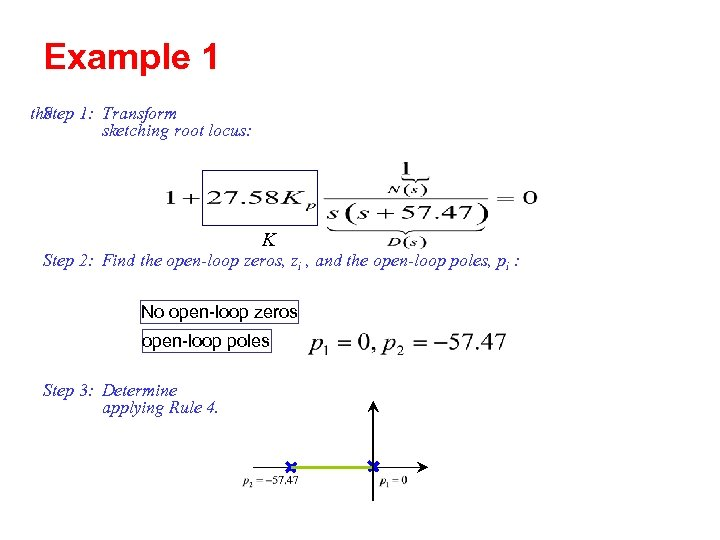Example 1 the 1: Transform Step sketching root locus: K Step 2: Find the open-loop zeros, zi , and the open-loop poles, pi : No open-loop zeros open-loop poles Step 3: Determine applying Rule 4.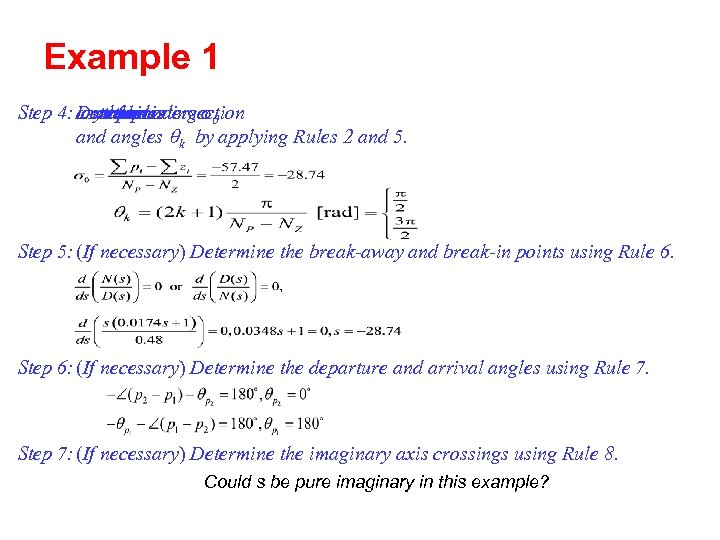Example 1 Step 4: Determine asymptotes corresponding s 0 numberintersection the ofand the and angles qk by applying Rules 2 and 5. Step 5: (If necessary) Determine the break-away and break-in points using Rule 6. Step 6: (If necessary) Determine the departure and arrival angles using Rule 7. Step 7: (If necessary) Determine the imaginary axis crossings using Rule 8. Could s be pure imaginary in this example?Example 1 Step 8: Use the information from Steps 1 -7 and Rules 1 -3 to sketch the root locus. Img. Axis 30 20 10 -57. 47 Real Axis -60 -28. 74 -50 -40 -30 -20 -10 0 0 -10 -20 -30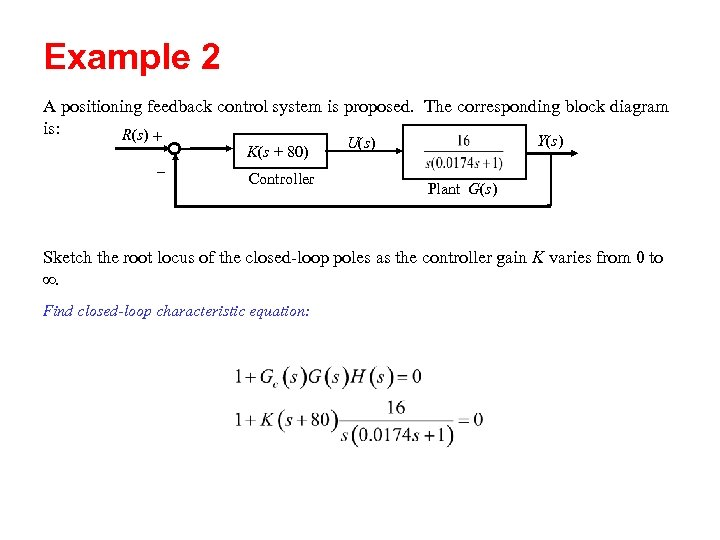Example 2 A positioning feedback control system is proposed. The corresponding block diagram is: R(s) + K(s + 80) - Controller Y(s) U(s) Plant G(s) Sketch the root locus of the closed-loop poles as the controller gain K varies from 0 to . Find closed-loop characteristic equation: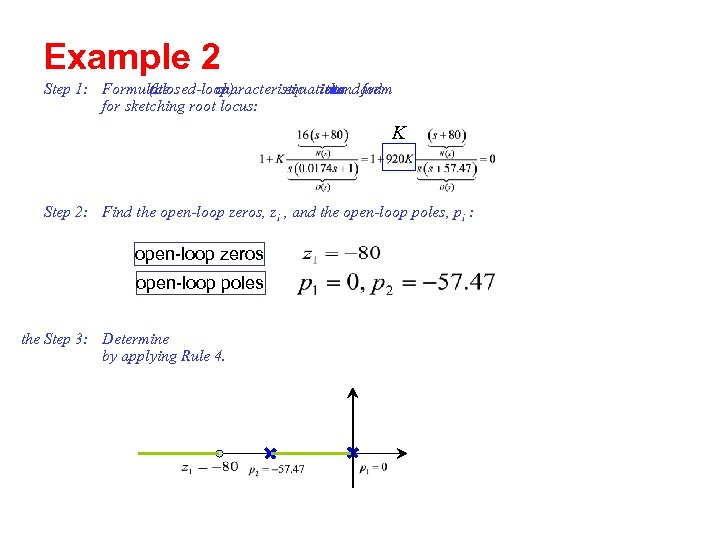Example 2 Step 1: Formulate (closed-loop) the characteristic into form equation standard the for sketching root locus: K Step 2: Find the open-loop zeros, zi , and the open-loop poles, pi : open-loop zeros open-loop poles the Step 3: Determine by applying Rule 4.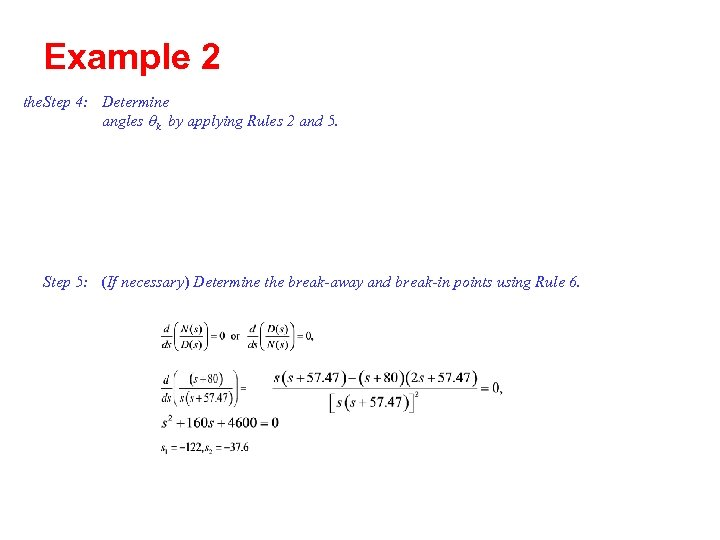Example 2 the Step 4: Determine angles qk by applying Rules 2 and 5. Step 5: (If necessary) Determine the break-away and break-in points using Rule 6.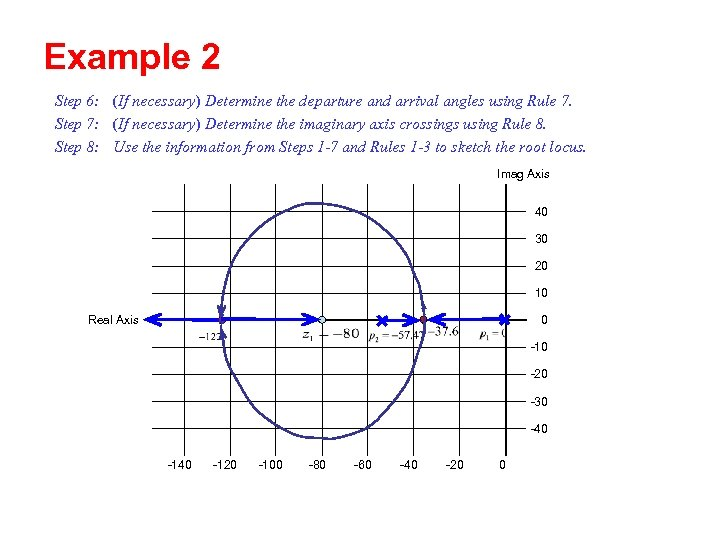Example 2 Step 6: (If necessary) Determine the departure and arrival angles using Rule 7. Step 7: (If necessary) Determine the imaginary axis crossings using Rule 8. Step 8: Use the information from Steps 1 -7 and Rules 1 -3 to sketch the root locus. Imag Axis 40 30 20 10 0 Real Axis -10 -20 -30 -40 -120 -100 -80 -60 -40 -20 0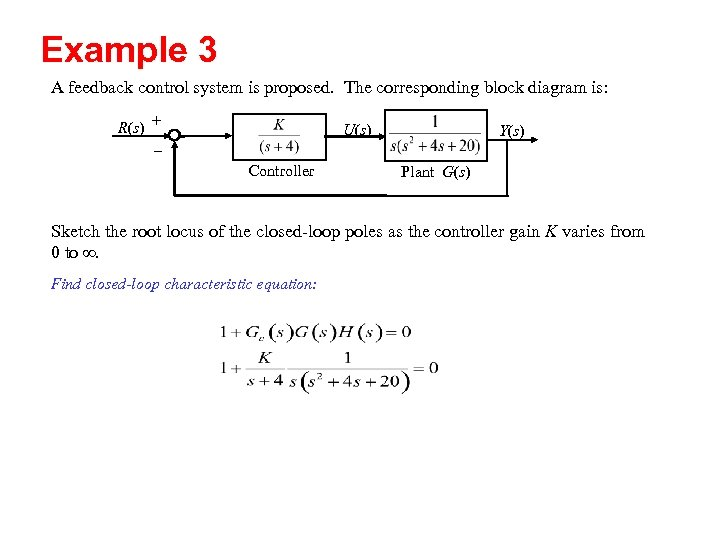Example 3 A feedback control system is proposed. The corresponding block diagram is: R(s) + - U(s) Controller Y(s) Plant G(s) Sketch the root locus of the closed-loop poles as the controller gain K varies from 0 to . Find closed-loop characteristic equation: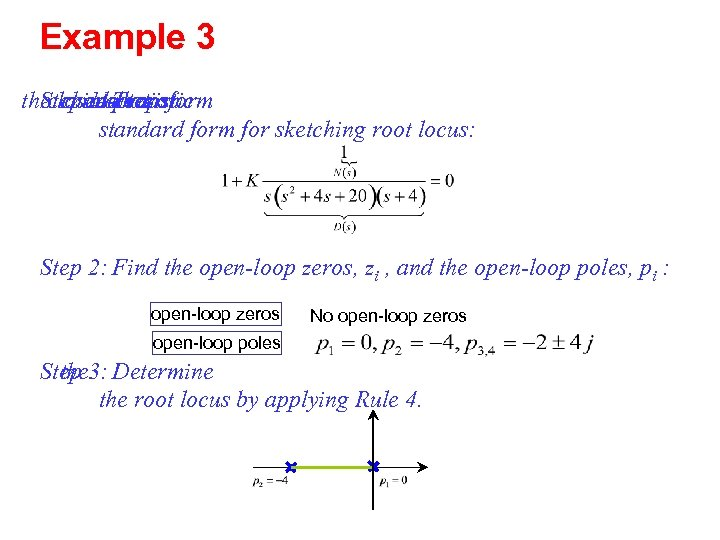Example 3 theclosed-loop Stepintothe characteristic 1: Transform equation standard form for sketching root locus: Step 2: Find the open-loop zeros, zi , and the open-loop poles, pi : open-loop zeros No open-loop zeros open-loop poles Step 3: Determine the root locus by applying Rule 4.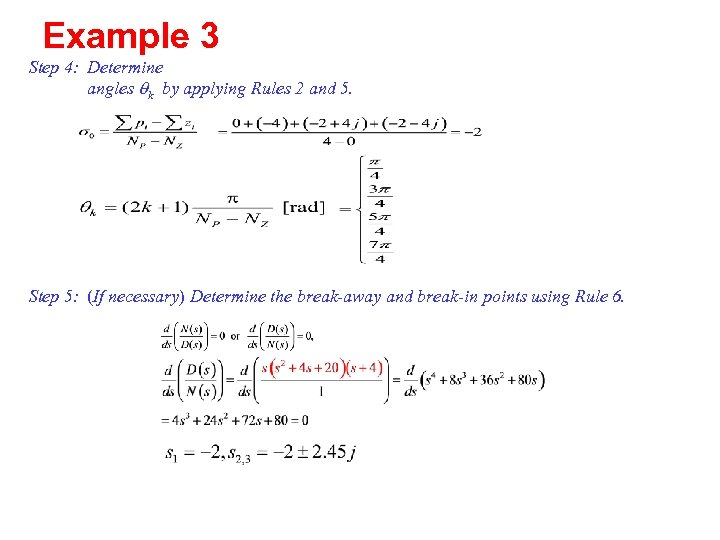Example 3 Step 4: Determine angles qk by applying Rules 2 and 5. Step 5: (If necessary) Determine the break-away and break-in points using Rule 6.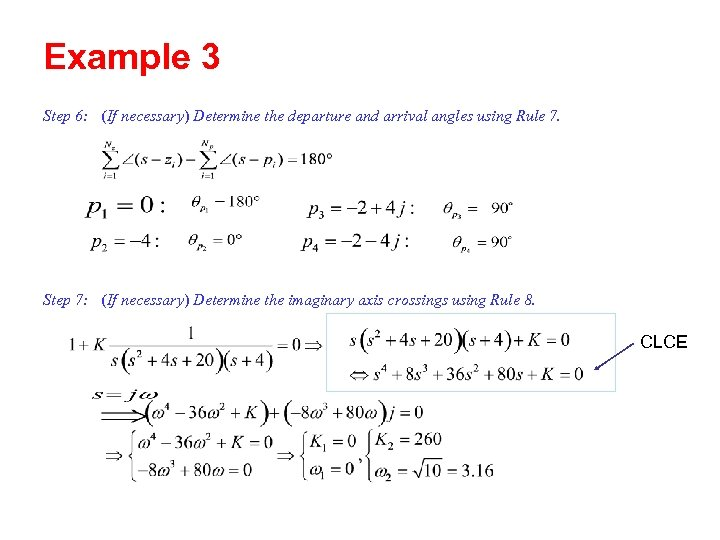Example 3 Step 6: (If necessary) Determine the departure and arrival angles using Rule 7. Step 7: (If necessary) Determine the imaginary axis crossings using Rule 8. CLCE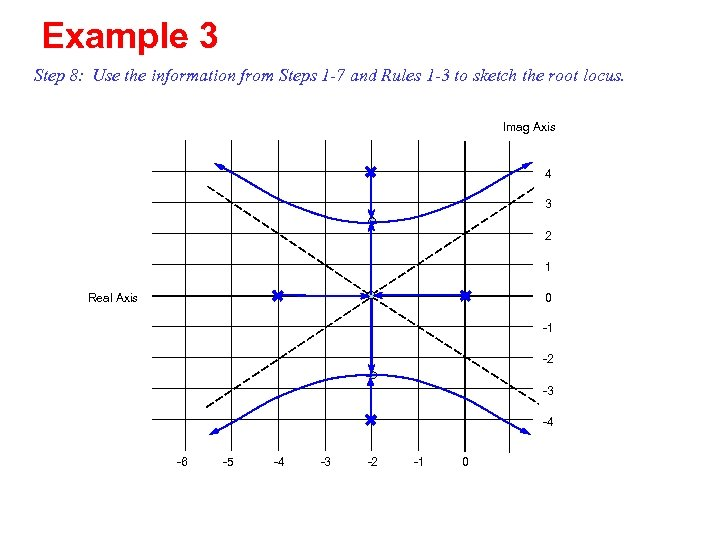Example 3 Step 8: Use the information from Steps 1 -7 and Rules 1 -3 to sketch the root locus. Imag Axis 4 3 2 1 Real Axis 0 -1 -2 -3 -4 -6 -5 -4 -3 -2 -1 0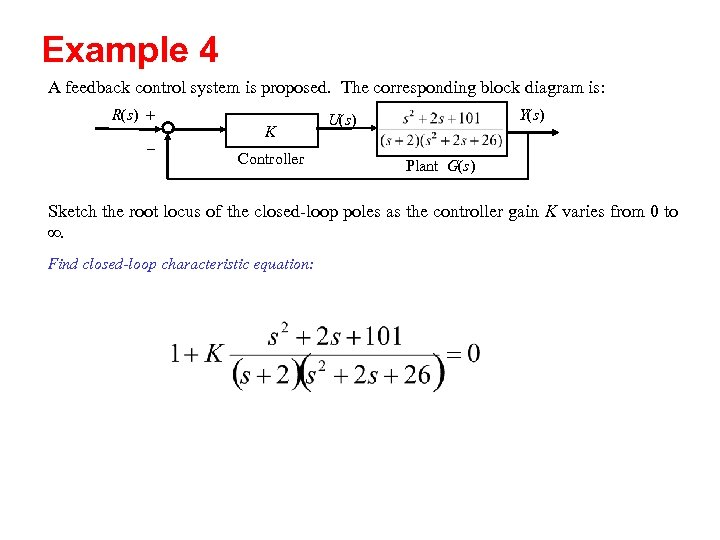Example 4 A feedback control system is proposed. The corresponding block diagram is: R(s) + - K Controller Y(s) U(s) Plant G(s) Sketch the root locus of the closed-loop poles as the controller gain K varies from 0 to . Find closed-loop characteristic equation: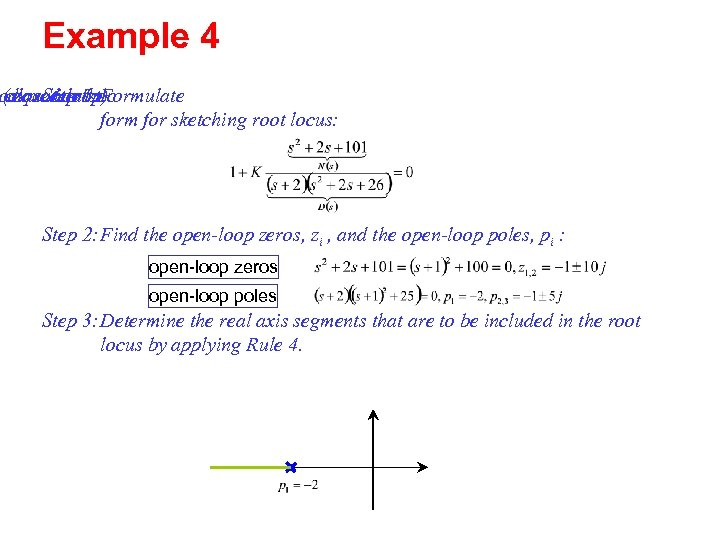Example 4 o equationthe (closed-loop) characteristic Step 1: Formulate form for sketching root locus: Step 2: Find the open-loop zeros, zi , and the open-loop poles, pi : open-loop zeros open-loop poles Step 3: Determine the real axis segments that are to be included in the root locus by applying Rule 4.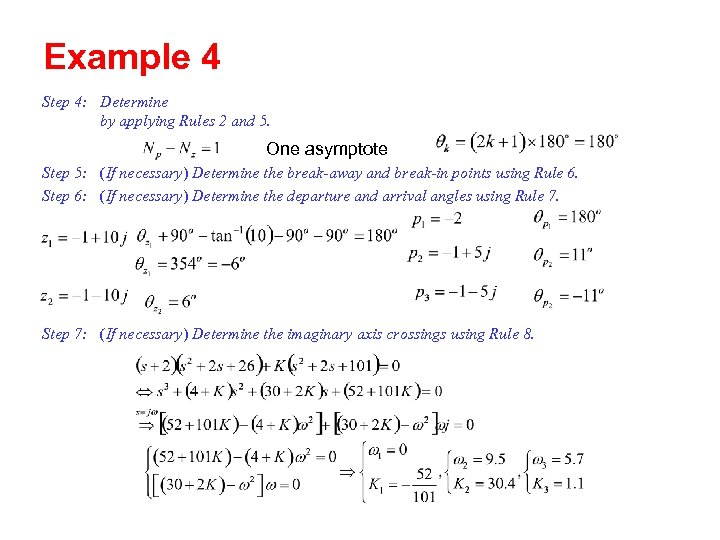Example 4 Step 4: Determine by applying Rules 2 and 5. One asymptote Step 5: (If necessary) Determine the break-away and break-in points using Rule 6. Step 6: (If necessary) Determine the departure and arrival angles using Rule 7. Step 7: (If necessary) Determine the imaginary axis crossings using Rule 8.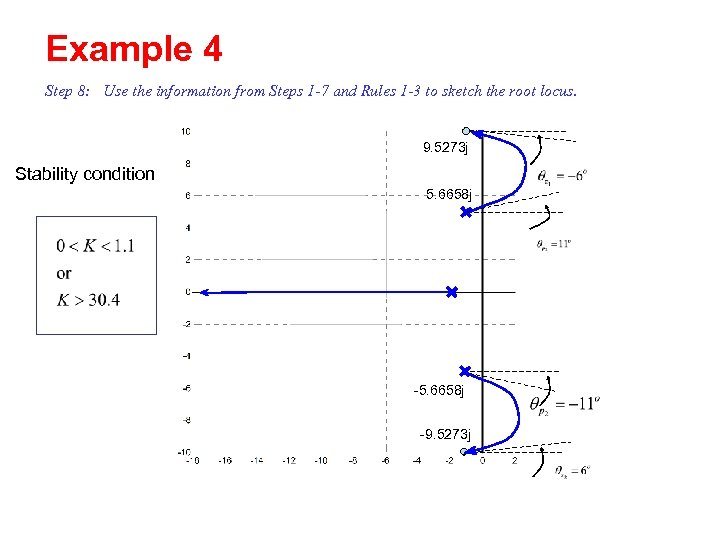Example 4 Step 8: Use the information from Steps 1 -7 and Rules 1 -3 to sketch the root locus. 9. 5273 j Stability condition 5. 6658 j -9. 5273 j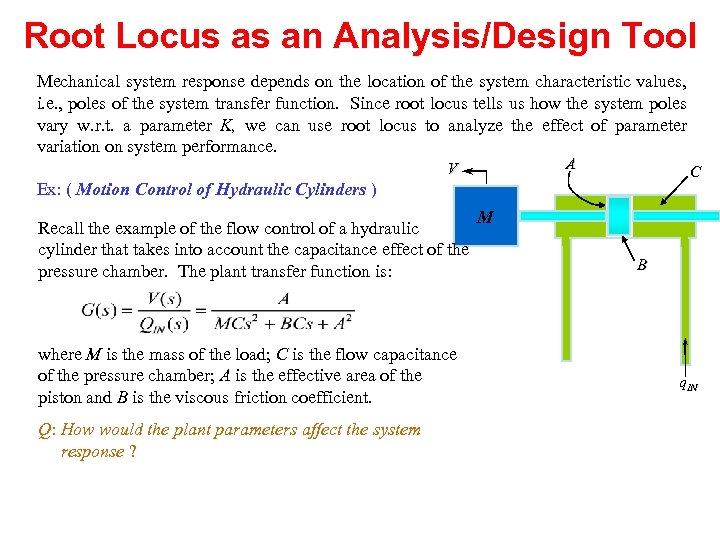Root Locus as an Analysis/Design Tool Mechanical system response depends on the location of the system characteristic values, i. e. , poles of the system transfer function. Since root locus tells us how the system poles vary w. r. t. a parameter K, we can use root locus to analyze the effect of parameter variation on system performance. A V C Ex: ( Motion Control of Hydraulic Cylinders ) Recall the example of the flow control of a hydraulic cylinder that takes into account the capacitance effect of the pressure chamber. The plant transfer function is: where M is the mass of the load; C is the flow capacitance of the pressure chamber; A is the effective area of the piston and B is the viscous friction coefficient. Q: How would the plant parameters affect the system response ? M B q. IN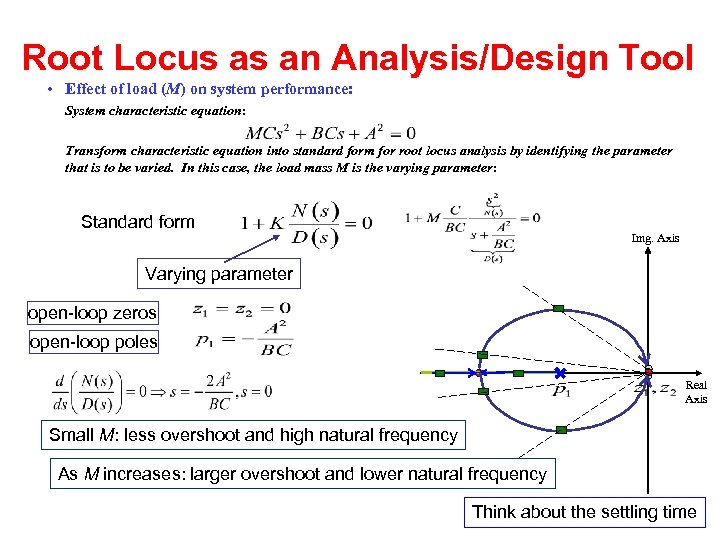Root Locus as an Analysis/Design Tool • Effect of load (M) on system performance: System characteristic equation: Transform characteristic equation into standard form for root locus analysis by identifying the parameter that is to be varied. In this case, the load mass M is the varying parameter: Standard form Img. Axis Varying parameter open-loop zeros open-loop poles Real Axis Small M: less overshoot and high natural frequency As M increases: larger overshoot and lower natural frequency Think about the settling time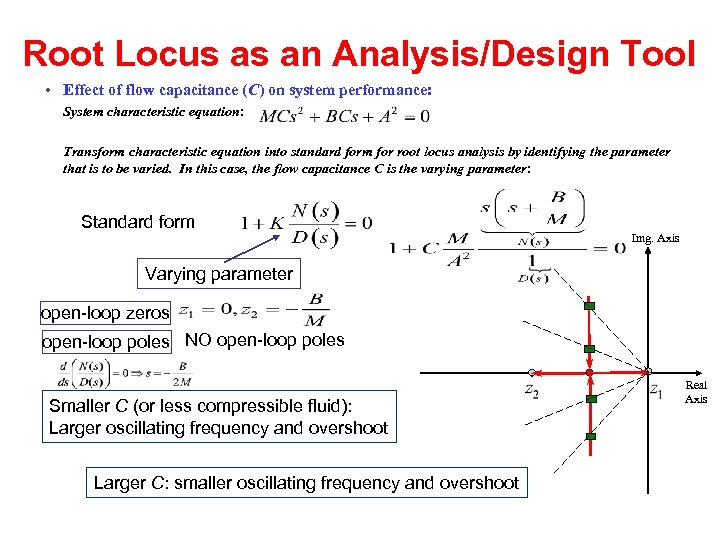Root Locus as an Analysis/Design Tool • Effect of flow capacitance (C) on system performance: System characteristic equation: Transform characteristic equation into standard form for root locus analysis by identifying the parameter that is to be varied. In this case, the flow capacitance C is the varying parameter: Standard form Img. Axis Varying parameter open-loop zeros open-loop poles NO open-loop poles Smaller C (or less compressible fluid): Larger oscillating frequency and overshoot Larger C: smaller oscillating frequency and overshoot Real Axis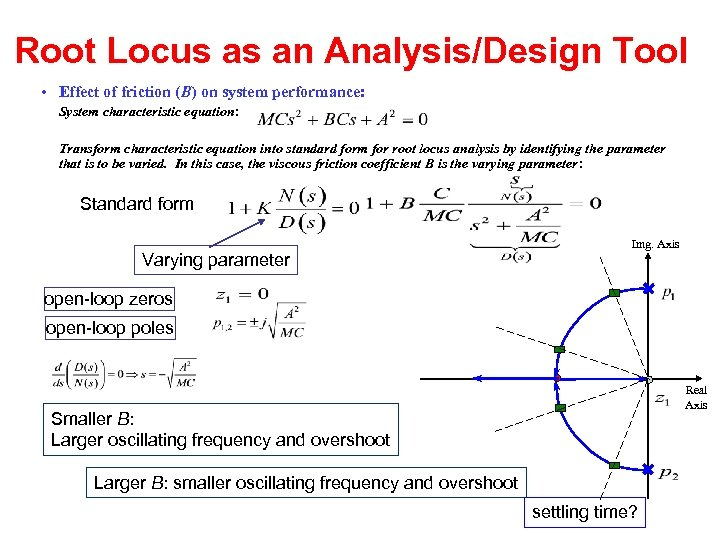Root Locus as an Analysis/Design Tool • Effect of friction (B) on system performance: System characteristic equation: Transform characteristic equation into standard form for root locus analysis by identifying the parameter that is to be varied. In this case, the viscous friction coefficient B is the varying parameter: Standard form Varying parameter Img. Axis open-loop zeros open-loop poles Real Axis Smaller B: Larger oscillating frequency and overshoot Larger B: smaller oscillating frequency and overshoot settling time?